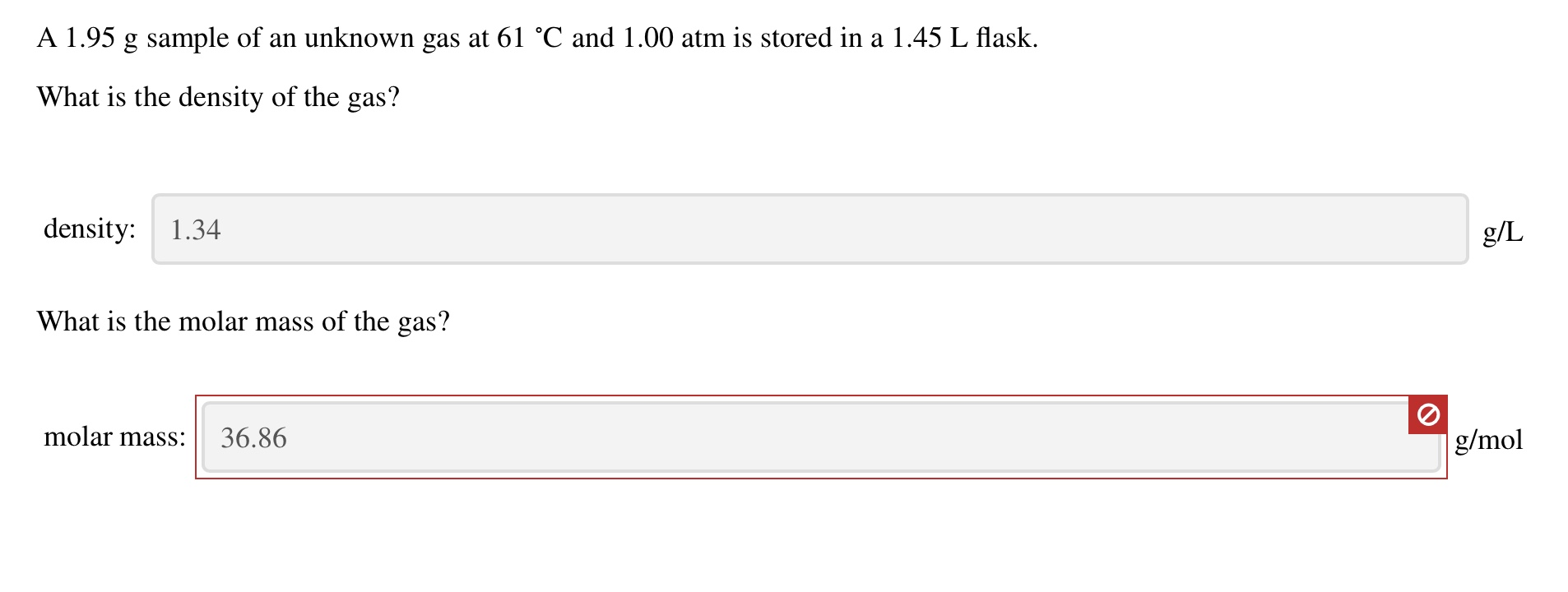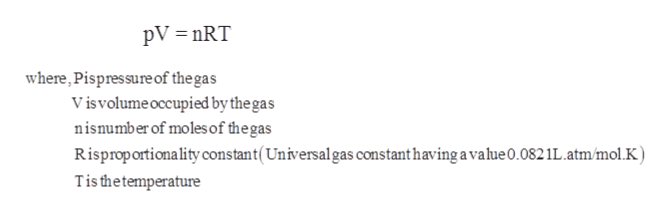# A 1.95 g sample of an unknown gas at 61 °C and 1.00 atm is stored in a 1.45 L flask.What is the density of the gas?density: 1.34g/LWhat is the molar mass of the gas?molar mass:36.86g/mol

Question
192 views
A 1.95 g sample of an unknown gas at 61 ∘C and 1.00 atm is stored in a 1.45 L flask.

What is the molar mass of the gas?help_outlineImage TranscriptioncloseA 1.95 g sample of an unknown gas at 61 °C and 1.00 atm is stored in a 1.45 L flask. What is the density of the gas? density: 1.34 g/L What is the molar mass of the gas? molar mass: 36.86 g/mol fullscreen
check_circle

star
star
star
star
star
1 Rating
Step 1

Given information:

Mass of sample = 1.95 g

Temperature = 61 °C

Pressure of gas = 1.00 atm

Volume of flask = 1.45 L

Step 2

Ideal gas law gives the empirical relationship between pressure, volume, temperature, and amount of gas that is number of moles of a gas. The ideal gas equation is represented as follows:help_outlineImage TranscriptionclosepV nRT where,Pispressure of the gas Visvolumeoccupied by the gas nisnumber of moles of the gas Risproportionality constant(Universalgas constant having a value 0.082 1L. atm/mol.K) Tis thetemperature fullscreen
Step 3

As, the temperature of gas is given in degree Celsius so,...

### Want to see the full answer?

See Solution

#### Want to see this answer and more?

Solutions are written by subject experts who are available 24/7. Questions are typically answered within 1 hour.*

See Solution
*Response times may vary by subject and question.
Tagged in

### Gas laws